# Efficient Generation of Dynamic Pulses

September 8, 2021 by Andrea Corna

Solid-state qubits are typically characterized and operated by a series of very short pulses. To achieve a high-fidelity control, it is necessary to generate many sequences of them, with very accurate timing.

In this blog post, I will show how to use the advanced feature set of the HDAWG Arbitrary Waveform Generator and the SHFSG Signal Generator to generate efficiently both simple and complex sequences. Their parameters can be adjusted dynamically, to have an efficient workflow, but also to realize closed-loop feedback experiments.

## Amplitude Sweep Rabi Experiment

Let’s start by the one of the simplest characterization experiments: the measurement of Rabi oscillations. This experiment is often used to kickstart measurement of qubits, since it can demonstrate that the device under test is indeed a two-level system (i.e., a qubit) and that it can be controlled coherently. Moreover, it can be used to determine the basic parameters to control the qubit, like resonance frequency, pulse duration and amplitude to perform a π rotation .

A very common variant in the class of Rabi experiments is the so-called Amplitude Rabi. The control pulse shape, length and frequency are kept constant, while the amplitude is varied, so the pulse energy. The expected outcome is an oscillation of the average population of the two levels involved in the transition. Such frequency of oscillation is the Rabi frequency. By keeping the pulse length constant, we also keep the bandwidth of the pulse the same, so we minimize the risk of exciting unwanted transitions. This is especially relevant for systems like artificial atoms that have a multitude of states, and we want to work only with two of them and realize a qubit.

How could we generate such pulses with the HDAWG or the SHFSG in an optimal way? The solution is in the command table (CT). The command table allows you to group waveform playback instructions with other timing-critical phase and amplitude setting commands in a single instruction. As the name implies, it’s a table that can be filled with many parameters, relative to the control of waveform playback.

Let’s construct now our amplitude Rabi. First, we need to declare the waveform that represent the envelope of our pulse in the sequence:


//Waveform definition
wave pulse = gauss(PULSE_TOTAL_LEN, PULSE_TOTAL_LEN/2, PULSE_WIDTH_SAMPLE);

and then we can assign an explicit index to this waveform, so we can use it in the command table:


assignWaveIndex(1,pulse, INDEX);

Here we declared only the envelope, other static parameters like the frequency modulation are set externally in Python.

Averaging is very important in a Rabi sequence, since we are interested in the average state population. We have two options how to average: sequential or cyclic. In the first case, we acquire all the results for an amplitude before moving to the next one. In the second, we sweep all the amplitudes one after the other, and then we repeat such sequence many times.

For the sequential case, it would look like this: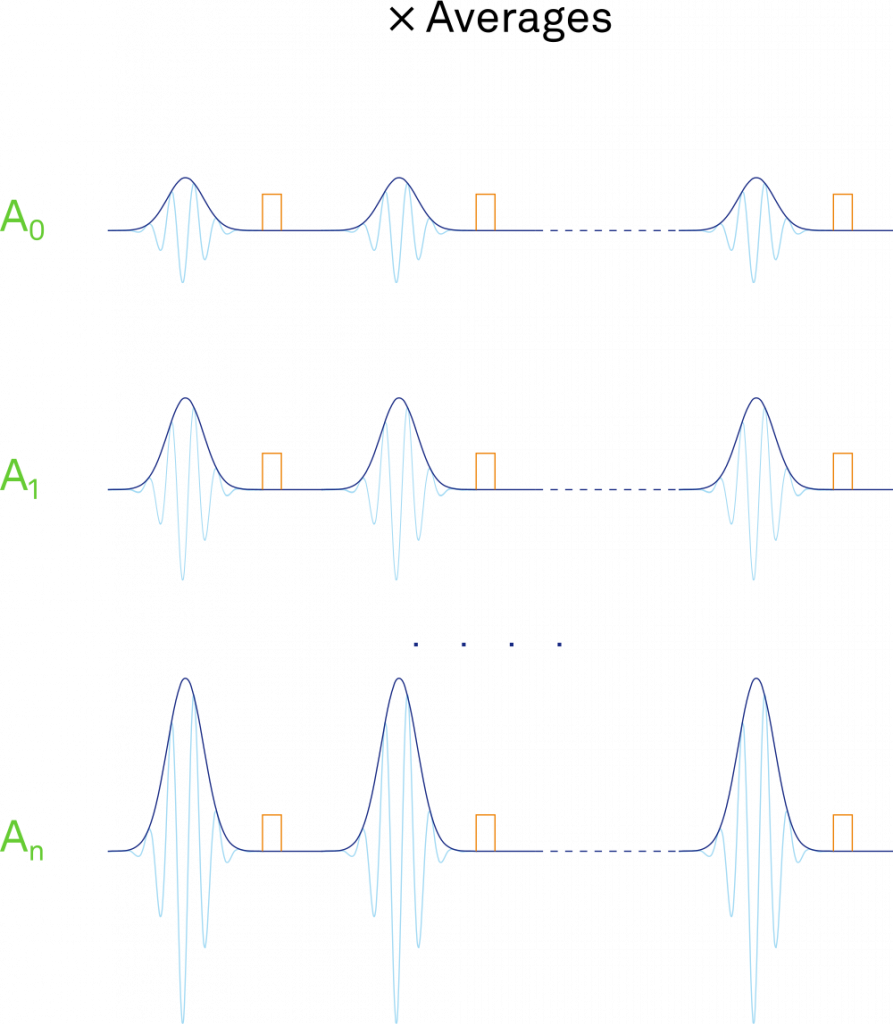while a cyclic averaging would be as follows: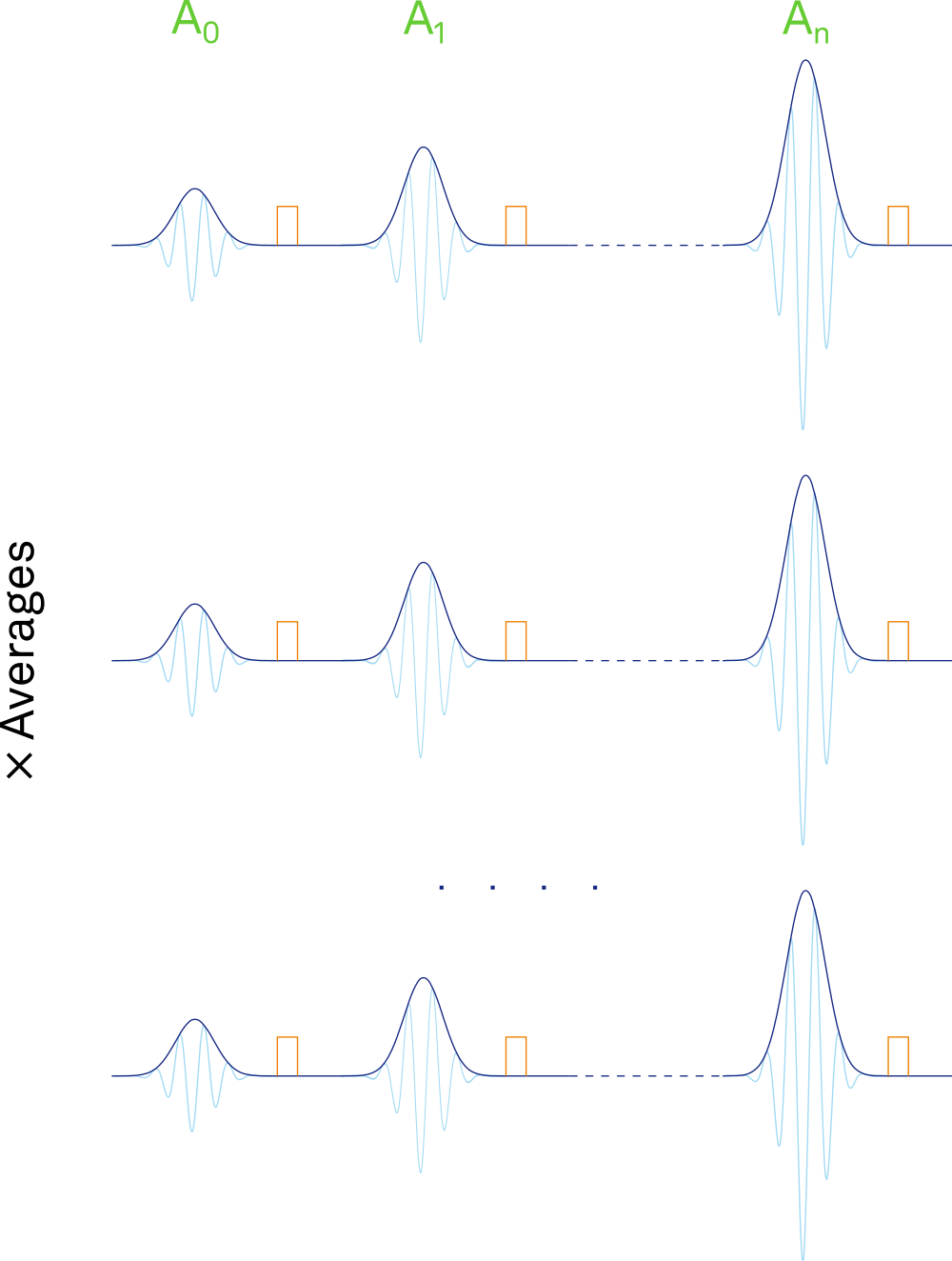To implement the sequential averaging, we need thecommand table programmed in this way[a][b]: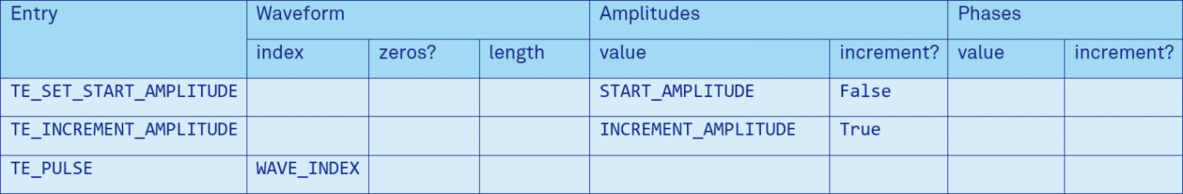and the relative sequence:


//Execution, sequential average
//Set start amplitude
executeTableEntry(TE_SET_START_AMPLITUDE);
repeat (NUM_AMPLITUDES) {
repeat (NUM_AVERAGES) {
//Reset the oscillator (not really needed for Rabi)
resetOscPhase();
//Play pulse
executeTableEntry(TE_PULSE);

}

//Increment amplitude
executeTableEntry(TE_INCREMENT_AMPLITUDE);
}

As we can see, we have two commands respectively to set the start amplitude and to increment (or decrement) it. A third entry play the pulse with such amplitude.

In this way, we are very efficient with respect to the waveform memory usage: we defined only one waveform and we can generate thousands or millions of pulses in a single sequence just reusing it! The amplitude increment, and the number of iterations in the two loops can be small or large and the sequence memory would not change. So, we can make very fine and time-consuming sweeps efficiently, without reloading the waveform or the sequence to step over the next value.

Of course, it’s possible to combine waveform play and amplitude changes; the update of the amplitude parameter will happen just at the start of the waveform playback. That is useful for the cyclic averaging version: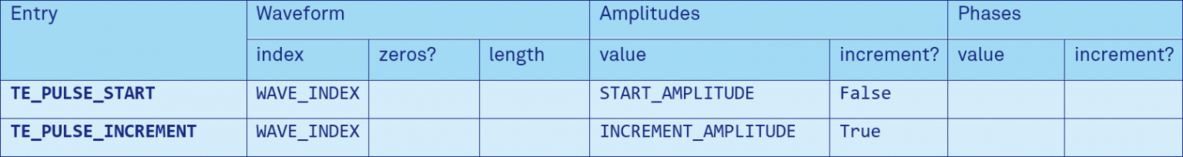and here the sequence would be:


//Execution, cyclic average
//Averaging loop
repeat (NUM_AVERAGES) {
//Reset the oscillator (not really needed for Rabi)
resetOscPhase();
//Play start pulse
executeTableEntry(TE_PULSE_START);

repeat (NUM_AMPLITUDES-1) {
//Reset the oscillator (not really needed for Rabi)
resetOscPhase();
//Play pulse and increment amplitude
executeTableEntry(TE_PULSE_INCREMENT);

}
}

## Ramsey Experiment

Typically, the next step is to further calibrate and characterize the qubit with a Ramsey sequence.

In this sequence, we have two π/2 pulses, separated by a wait time where the qubit evolves. The first π/2 pulse brings the qubit in a superposition of the ground and excited state. Then, it freely evolves for a time τ by precessing around the Z axis. Finally, a second π/2 pulse bring the state either to the ground or excited state, depending on the phase accumulated during the free evolution phase. By varying the time τ, it’s possible to evaluate the decoherence time T2* of the qubit.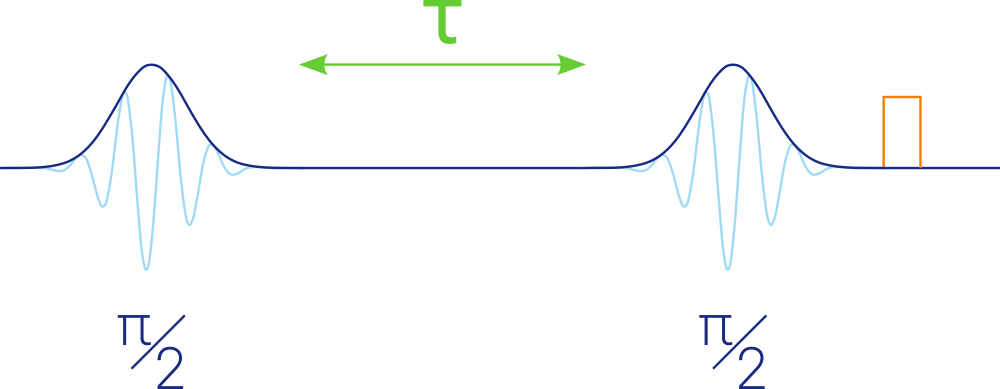We could construct this sequence easily (averaging omitted for simplicity):


var t = T_START;
do {
//Reset the oscillator
resetOscPhase();

//Play first pulse
playWave(1,pulse);
//Evolution time t
playZero(t);
//Play second pulse
playWave(1,pulse);

//Increase wait time
t += T_STEP;

//Loop until the end
} while (t < T_END);                          

In this sequence, we used the special command playZero. It plays a series of samples equal to zero, with the big advantages that no waveform memory is used and that the length can be a dynamic variable. So, the evolution time can assume many values and it’s arbitrarily long.

This sequence works as expected, but it suffers of a quite important limitation. In fact, the argument of playZero need to be a multiple of 16, like the granularity of the waveforms for playWave. If the sample rate is the maximum of 2.4 GSa/s that mean a minimal step size of 6.66 ns, which may be unsuitable for many high-precision experiments. Luckily, the command table it’s here to solve the issue!

How to do so? We split the wait time in two parts, a coarse and a fine. The coarse one is handled by playZero as before.

For the fine one, we create sixteen copies of the pulse, each one shifted by one sample more and padded on the right to respect the granularity and have constant length:


//Create shifted waveforms
cvar j;
for (j = 0; j < 16; j++) {
//Create the j-samples shifted waveform
wave w_shifted = join(zeros(j), pulse, zeros(16-j));
assignWaveIndex(1, w_shifted, j);
}

which would create something like this: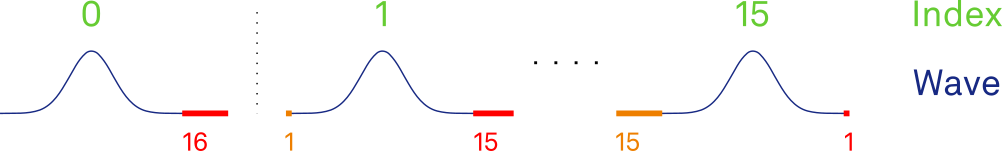Then, we reference them in the command table with a one-to-one mapping: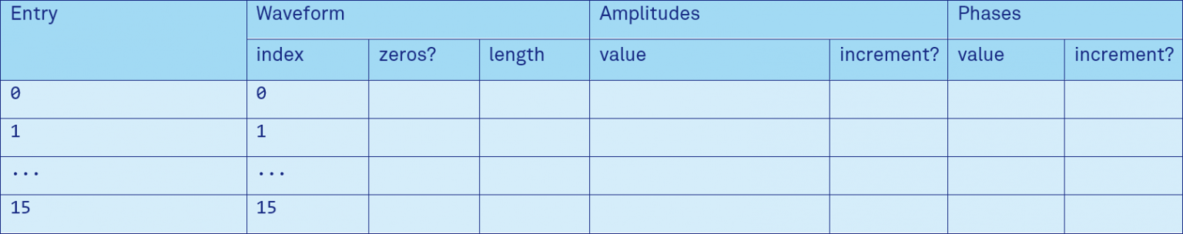By playing the correct command table entry as second pi/2 pulse, we can adjust the delay. The playback would look like this: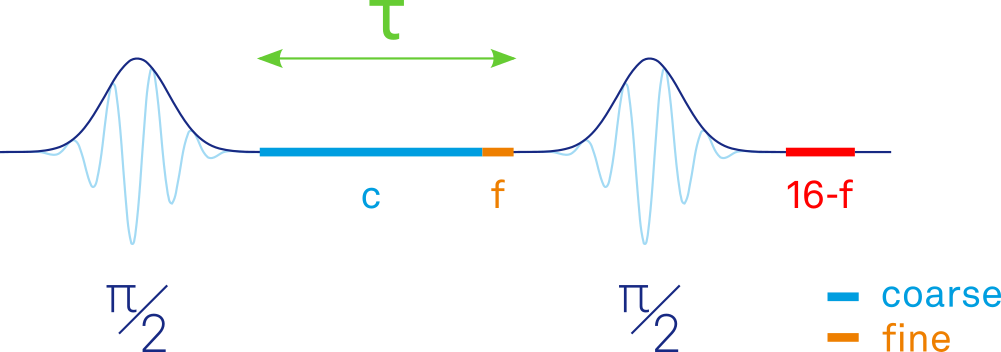How do we execute sequence? The fine delay can be calculated by taking the four least significant bits of the evolution time; this value can be used as argument to the command table execution. The instruction playZero automatically discards the fine part, so we can just pass the original wait time as argument. However, we need to execute playZero only if its argument is bigger than 16 (the minimum playZero length)[c].
The sequence will look like this[d]:


//Execution, no averaging
var t = T_START;
var t_coarse, t_fine;
do {
//The fine shift is the four least significant bits
t_fine = t & 0xF;
//Check if coarse delay is needed (boolean).
//Equivalent to (t >= 16)
coarse = t & -0x10;

//Reset the oscillator
resetOscPhase();

//Play first pulse, no shift
playWave(1,pulse);
if(coarse)
playZero(t); //Evolution time t (coarse)

//Play second pulse, fine shift
executeTableEntry(t_fine);

//Increase wait time
t += T_STEP;

//Loop until the end
} while (t < T_END);                     

Now we have all the elements: we can sweep the evolution time with sample precision, arbitrary length, and minimal memory consumption, just for the waveform copies.

## Hahn Echo Experiment

The measured qubit decoherence time with a Ramsey sequence is affected by noise sources over a broad frequency spectrum. The spin echo technique is commonly used to cancel out the contributions from low frequencies and access the intrinsic decoherence time T2. This sequence originates from the field of NMR and broadly speaking spin qubits, where this is mostly due to the inhomogeneous magnetic field and coupling with remote spins. Similarly, for superconducting qubits, the major contribution to the decoherence time at low frequencies is due to the magnetic flux noise .

The sequence is very similar to the Ramsey sequence, with an additional π pulse between the two π/2 pulses. The random phases accumulated during the two evolution times cancel out, since the qubit will process in opposites directions due to the π pulse.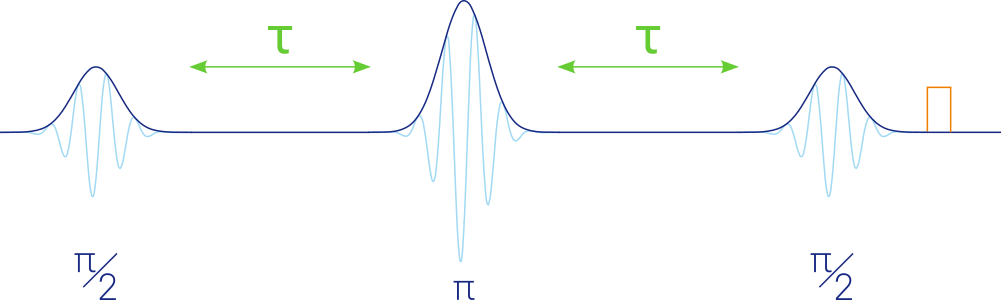As before, we can sweep the tau time with high precision but how do we calculate the fine/coarse parameters for the second pulse? Differently from the Ramsey sequence, now we have to take into account the right padding of the second pulse, that changes with the fine shifting. This can be easily done by subtracting the right padding of the second pulse to the target wait time between pulses 2 and 3. This quantity is equal to (16-fine1)[e].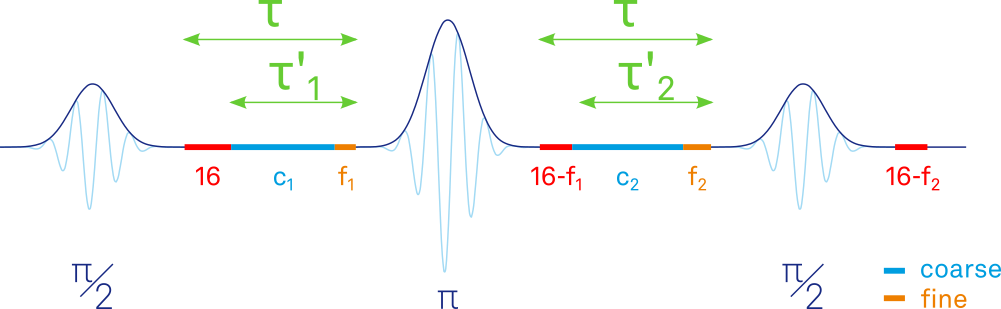We also need a different sets of command table entries for the π pulse. For simplicity, we assume an amplitude of 0.5 for the π/2 pulse and 1.0 for the π pulse: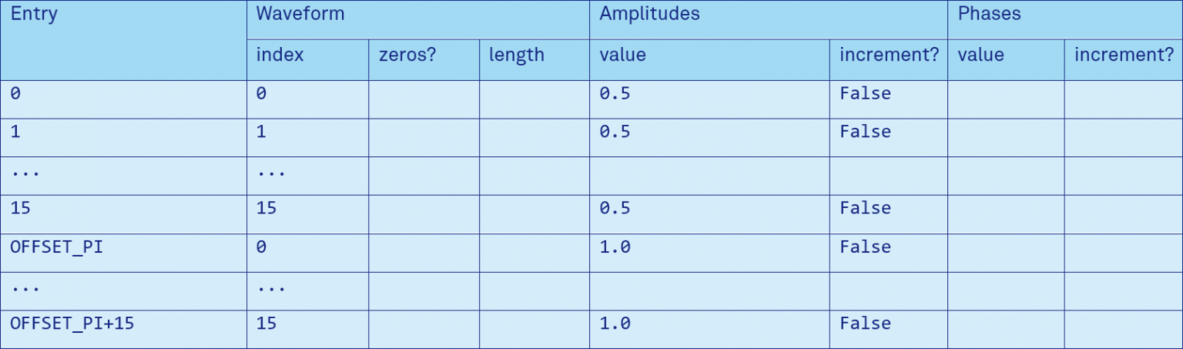Then we can proceed as before with the fine/coarse calculations:


//Calculate target tau (remove 16 for the right padding, common to all pulses)
tp = t - 16;

//The first delay doesn't need further adjustments, since the fine shift
// of the first pulse is always zero, so we use t1 = tp
//Fine shift 1 (samples), four least significant bits
t_fine1 = tp & 0xF;
//Fine shift 1 (CT entry index, adjust for pi-pulse)
t_fine1_entry = t_fine1 + OFFSET_PI;
//Check if coarse delay 1 is needed (boolean)
coarse1 = tp & -0x10;

//Calculate target tau for the second wait.
//Adjust for fine delay 1 by adding it to tp, so equal to
// t2 = t - (16 - t_fine1)
t2 = tp + t_fine1;
//Fine shift 2 (samples and CT entry, no offset for pi/2-pulse)
t_fine2 = t2 & 0xF;
//Check if coarse delay 2 is needed (boolean)
coarse2 = t2 & -0x10;

//Reset the oscillator
resetOscPhase();

//First pi/2-pulse, no shift
//Play first pulse, no fine shift
executeTableEntry(0);

//First tau wait time
if(coarse1)
//Evolution time tau (coarse)
playZero(tp);

//pi-pulse
//Play second pulse, fine shift
executeTableEntry(t_fine1_entry);

//Second tau wait time
if(coarse2)
//Evolution time tau (coarse)
playZero(t2);

//Second pi/2-pulse
//Play third pulse, fine shift
executeTableEntry(t_fine2);           

As before, we can sweep the evolution time with sample precision, arbitrary length, and minimal memory consumption, but for a more complex sequence.

## Back to Rabi, Length Sweep Version

In the Rabi experiment before, we swept the amplitude of the pulse. An alternative is to keep the amplitude constant and sweep the pulse length instead. In principle the two methods are equivalent since the goal is to sweep the pulse energy. Controlling the pulse length can be useful to observe more oscillation, while with the amplitude we have a smaller range of control. It’s also useful for qubits with modest Rabi frequency. Moreover, we don’t have to worry about the linearity of the mixer used for upconversion at high power.

For this experiment we use a so-called flat-top pulse. It’s composed by rise and fall sections and in the middle a constant amplitude that can be varied.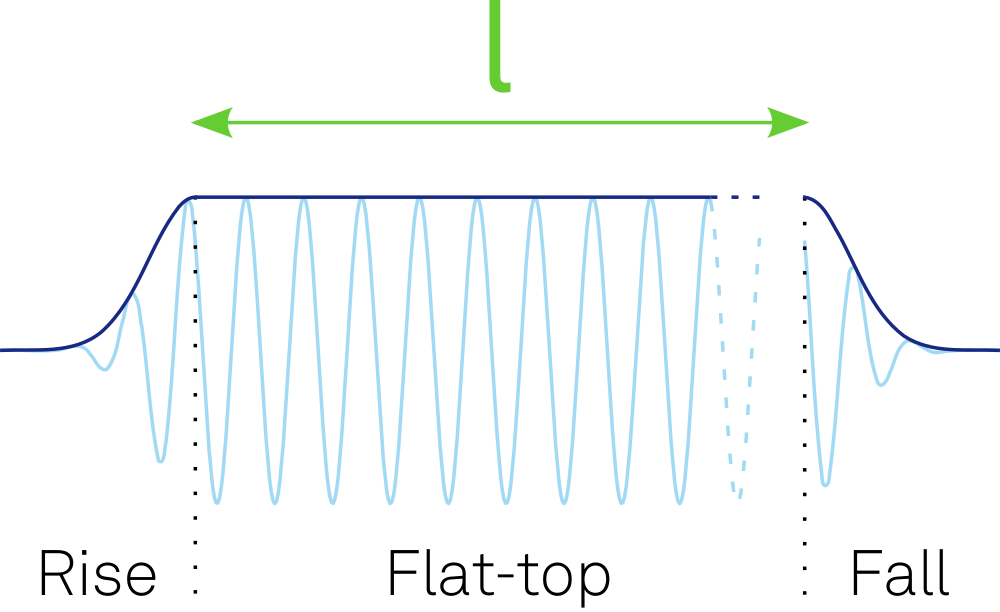Compared to a square pulse, it minimizes the bandwidth requirement of the hard edges. And in contrast to a pulse like a varying width Gaussian, it maximizes the pulse power.

This experiment can be realized in the same way as the Ramsey experiment. First, we have to change the two pulses with the rise/fall edges. We need one waveform for the rising edge, and 16 shifted copies of the falling edge. The left padding now will be the maximum of the waveform, instead of zeros: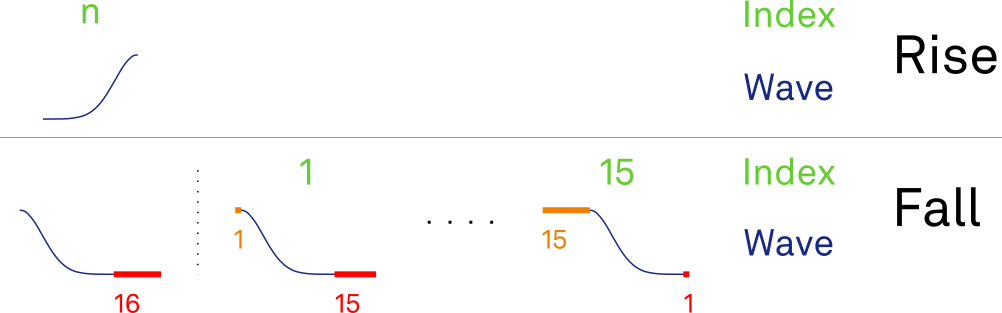If we use Gaussian edges, they can be created like this[f]:


//Waveform definition
wave pulse = gauss(PULSE_TOTAL_LEN,
AMPLITUDE,PULSE_TOTAL_LEN/2,
PULSE_WIDTH_SAMPLE);
wave w_rise = cut(pulse, 0, PULSE_TOTAL_LEN/2-1);
wave w_fall = cut(pulse, PULSE_TOTAL_LEN/2,
PULSE_TOTAL_LEN-1);

//Create shifted fall waveforms
cvar j;
for (j = 0; j < 16; j++) {
//Create the j-samples shifted waveform
wave w_shifted = join(rect(j, AMPLITUDE), w_fall, zeros(16-j));
assignWaveIndex(1, w_shifted, j);
}

and a command table identical to the Ramsey one.

Then we have to change the behaviour of playZero, to keep the last value of a played waveform instead of just outputting zeros. For that we have the hold option (node awgs/n/outputs/m/hold to True). Note that the AWG hold the last waveform sample, not the actual output, so that affect the envelope, not the modulation, as desired.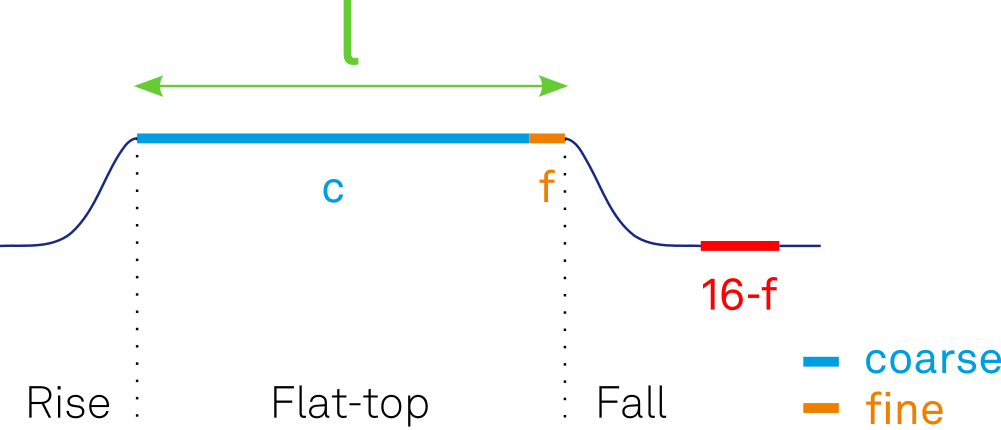Except for these modifications, the sequence is almost identical to the Ramsey experiment:


//The fine shift is the four least significant bits
t_fine = t & 0xF;
//Check if coarse delay is needed (boolean). Equivalent to (t >= 16)
coarse = t & -0x10;

//Reset the oscillator
resetOscPhase();

//Play rising edge
playWave(1,w_rise);
if(coarse)
//keep playing last value (needs awgs/n/outputs/*/hold = True)
playZero(t);

//Play falling edge, with fine shift
executeTableEntry(t_fine);           

As in the case of the Ramsey sequence, we can generate a pulse with variable width and sample precision without limitation on its length!

## Two-Axis Control

All the experiment we analyzed so far only dealt with the control of the qubit along the X axis of the rotating frame. One way to control the phase of the qubit, is to introduce a relative phase change to a control pulse, also called Virtual Z-gate . The simplest experiment is a modified Ramsey, with fixed evolution time (or even none) and a phase change on the second π/2 pulse: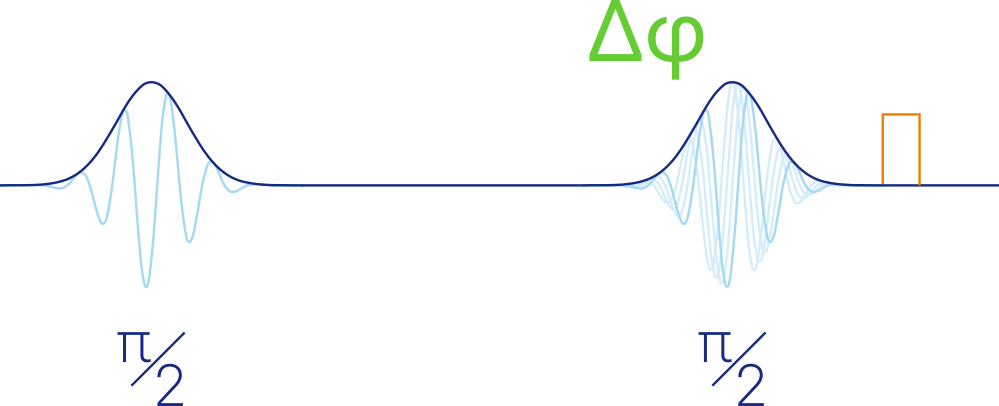The most efficient way to implement that is to use the command table, now with the phase field. The phase changes are instantaneous and aligned with the pulse edge.

Let’s define two waveforms, one for the first pulse, and another identical, but with the right fine shift


//Waveform definition
wave pulse_1 = gauss(PULSE_TOTAL_LEN,
PULSE_TOTAL_LEN/2,
PULSE_WIDTH_SAMPLE);
wave pulse_2 = join(zeros(T_FINE), pulse_1, zeros(16-T_FINE));
assignWaveIndex(1, pulse_1, FIRST_PULSE_INDEX);
assignWaveIndex(1, pulse_2, SECOND_PULSE_INDEX);

Then, our command table would be[g]: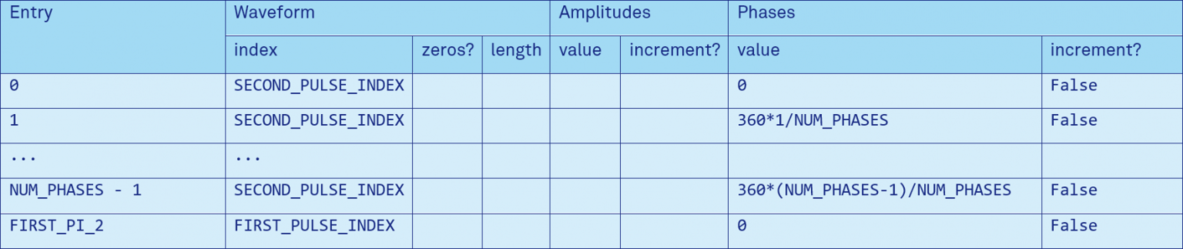with the sequence:


//Execution, no averaging
var phase = 0;
do {
//Reset the oscillator
resetOscPhase();

//Play first pulse, no shift, zero phase
executeTableEntry(FIRST_PI_2);
//Evolution time t (coarse)
playZero(T_COARSE);
//Play second pulse, fixed fine shift and added phase
executeTableEntry(phase);

//Increase the phase by 2PI/NUM_PHASES
phase += 1;

//Loop until the end
} while (phase < NUM_PHASES);            

This is the easiest example of two-axis control, but it can be extended to more complex algorithm, without dealing with many waveforms for each phase.

## Dynamic Control

Until now, we just performed linear sweeps of one quantity. Second or third sweep axes can be easily added on the controlling computer, without minimal performance overhead, as the first axis is the time-critical one.

What about a closed-loop feedback experiment, where we need to continuously change a parameter in a non-linear order?

### Computer-based approach

If we need a feedback loop that is simply fast to reduce the wall-clock time, we can use our computer to perform the calculation of the new parameter. This can give us enormous freedom in the computation, and we can have loops with few tens or hundreds of milliseconds of latency. This is particularly important in tuning and optimization algorithms, where a lot of sparse measurement points need to be probed quickly, but without the need to be real-time clock precise . In this case, we can’t afford to re-compile the sequence or update long the waveforms to change one or more parameter.

The solution for that are user registers. These are special variables that can exchange a value with the host computer, without the need to re-compile a sequence. If we have a sequence where we want to dynamically change the time t, we can acquire its value from the computer at the beginning of the sequence with:


var t = getUserReg(TIME_REGISTER);

and feed the sequencer with the intended values sequentially (in Python):


register_node = f'/{device:s}/awgs/{AWG_CORE:d}/userregs/{TIME_REGISTER:d}'
enable_node = f'/{device:s}/awgs/{AWG_CORE:d}/enable'

#Sequentially set all the time values
for v in time_values:
#Set User register
daq.setInt(register_node, v)

#Run the AWG and pool the status
daq.syncSetInt(enable_node, 1)

#Pool the sequencer status and wait until it’s done
while daq.getInt(enable_node) == 1:
time.sleep(0.005)

### Real-time approach

The user registers are a fast way of communicating with a computer, but it’s inherently non-real time. The OS decides dynamically how to distribute the CPU time, and the Ethernet network may fluctuate. When strict timing and low latency is needed, we need another solution.

The HDAWG can receive parameters in real-time on two interfaces: the Digital Input/Output interface (DIO) or the ZSync interface. The first one is useful when we connect directly to a UHFQA or when we have a custom microcontroller that we desire to communicate with. The second one is used together with the PQSC when we have a large system of Zurich Instrument devices and we need to communicate with them, for example to perform active qubit reset or complex quantum error correction experiments. They can also be used for multi-device synchronization, please refer to the HDAWG User Manual and the PQSC User Manual.

In the sequencer, we can fetch the last value sent over the interface[h] with


//Fetch last valid data received on the DIO
var t = getDIOTriggered();             

or with


//Fetch last valid data received from the PQSC
var t = getZSyncData(ZSYNC_DATA_TYPE); 

Differently from the user register, we can place this call in any point of our sequence since that will take only few clock cycles. getDIOTriggered always returns the raw data received over DIO, while getZSyncData can quickly process the received data according to the formula:


getZSyncData := ((zsync_data >> SHIFT) & MASK) + OFFSET

where SHIFT, MASK and OFFSET are constant defined in nodes settings. In this way, it’s possible to select a portion of the message (SHIFT and MASK) and target a specific portion of the command table (OFFSET).

If we don’t need any other custom treatment to the variable data and we need to be even faster, we can bypass the sequencer and feed this value directly to the command table. We could do that with the commands playWaveDIO and playWaveZSync. They are equivalent to these operations, even if way faster:


playWaveDIO := executeTableEntry(((getDIOTriggered() >> SHIFT) & MASK))
playWaveZSync := executeTableEntry(((zsync_data >> SHIFT) & MASK) + OFFSET)

## Conclusion

The HDAWG is a flexible tool for fast and accurate quantum measurements. Here we have shown how to perform efficient Rabi, Ramsey and Hahn Echo experiments, but many more algorithms can be implemented. The variety of the interface lets you to have dynamic control, either computer-based or real-time.

All the code presented here can be found in our GitHub space.

Did this post trigger your interest? Do you have a new and demanding experiment that you want to perform? Write to me at Andrea.Corna@zhinst.com, I’m looking forward to your challenge!

## Notes

1. ^Due to a limitation in LabOne 21.08, every entry needs an associated waveform to be effective; the easiest is 32 samples of zeros. The command table in the blog post doesn’t reflect that, while this fix is present in the code on GitHub.
2. ^For simplicity, here we considered only one amplitude value. When working with an external IQ mixer on the HDAWG or complex waveforms on the SHFSG, we have two identical amplitudes.
3. ^The fine time calculation and the coarse play condition are calculated before the sequence executes, to be sure these values are ready during the playback. For performance reasons, the decision to play a coarse delay, is evaluated as bitwise AND with an appropriate mask.
4. ^Averaging removed for simplicity
5. ^Differently from the Ramsey sequence, we do this adjustment also for the target wait time between pulses 1 and 2, as for symmetry now we play the pulse with the right padding. of 16 samples So, we always subtract 16 samples from the target time
6. ^Due to a limitation in LabOne 21.08, we need to add 8 samples padding both on the left and right of all samples. This limitation will be removed in a future version of LabOne. The snippet of code in the blog post doesn’t reflect that, while this fix is present in the code on GitHub.
7. ^For simplicity, here we considered only one phase. When working with an external IQ mixer on the HDAWG or complex waveforms on the SHFSG, we have two phases. The first one will be the same as here, and the second just 90° more.
8. ^The DIO interface needs to be configured to define the trigger condition when the data is sampled. For ZSync this is automatic. Please refer to the HDAWG User Manual.

## References

1. ^ Devoret, D. V. (2003). Rabi oscillations, Ramsey fringes and spin echoes in an electrical circuit. Fortschr. Phys.(51), 462-468. doi:10.1002/prop.200310063
2. ^ G. Ithier, E. C. (2005). Decoherence in a superconducting quantum bit circuit. Phys. Rev. B(72), 134519. doi:10.1103/PhysRevB.72.134519
3. ^ R. Maurand, X. J.-P. (2016). A CMOS silicon spin qubit. Nature Communications(7), 13575. doi:10.1038/ncomms13575
4. ^ David C. McKay, C. J. (2017). Efficient Z Gates for Quantum Computing. Phys. Rev. A(96), 022330. doi:10.1103/PhysRevA.96.022330.
5. ^ Anasua Chatterjee, F. A. (2021). Autonomous estimation of high-dimensional Coulomb diamonds from sparse measurements. arXiv preprint.
6. ^ H. Moon, D. T. (2020). Machine learning enables completely automatic tuning of a quantum device faster than human experts. Nature Communications(11), 4161. doi:10.1038/s41467-020-17835-9